# RD Sharma Solutions Class 6 Maths Chapter 4 Operations on Whole Numbers

Read RD Sharma Solutions Class 6 Maths Chapter 4 Operations on Whole Numbers below, students should study RD Sharma class 6 Mathematics available on Studiestoday.com with solved questions and answers. These chapter wise answers for class 6 Mathematics have been prepared by teacher of Grade 6. These RD Sharma class 6 Solutions have been designed as per the latest NCERT syllabus for class 6 and if practiced thoroughly can help you to score good marks in standard 6 Mathematics class tests and examinations

Exercise 4.1

Question 1:   Fill in the blanks to make each of the following a true statement:

(i) 359 + 476 = 476 + ____

(ii) ____ + 1952 = 1952 + 2008

(iii) 90758 + 0 = ____

(iv) 54321 + (489 + 699) = 489 + (54321 + ____)

Solution 1:

(i) 359 + 476 = 476 + 359 (Commutative Identity)

(ii) 2008 + 1952 = 1952 + 2008 (Commutative Identity)

(iii) 90758 + 0 = 90758 (Additive Identity)

(iv) 54321 + (489 + 699) = 489 + (54321 + 699) (Associative Identity)

Question 2:  Add each of the following and check by reversing the order of addends:

(i)       5628 + 39784

(ii)      923584 + 178

(iii)     15409 + 112

(iv)     2359 + 641

Solution 2:

(i) Addition: 5628 + 39784 = 45412

Check by reversing the orders: 39784 + 5628 = 45412

(ii) Addition: 923584 + 178 = 923762

Check by reversing the orders: 178 + 923584 = 923762

(iii) Addition: 15409 + 112 = 15521

Check by reversing the orders: 112 + 15409 = 15521

(iv) Addition:  2359 + 641 = 3000

Check by reversing the orders: 641 + 2359 = 3000

Question 3:  Determine the sum by suitable rearrangements:

(i)       953 + 407 + 647

(ii)      15409 + 178 + 591 + 322

(iii)     2359 + 10001 + 2641 + 9999

(iv)     1 + 2 + 3 + 4 + 1996 + 1997 + 1998 + 1999

(v)      10 + 11 + 12 + 13 + 14 + 15 + 16 + 17 + 18 + 19 + 20

Solution 3:

(i) 953 + 407 + 647 =

Therefore, 53 + 47 = 100

Therefore, (953 + 647) + 407

= 1600 + 407 = 2007

(ii) 15409 + 178 + 591 + 322=

409 + 91 = 500;

78 + 22 = 100

Therefore, (15409 + 591) + (178 + 322)

= (16000) + (500)

= 16500

(iii) 2359 + 10001 + 2641 + 9999=

59 + 41 = 100;

99 + 01 = 100

Therefore, (2359 + 2641) + (10001 + 9999)

= (5000) + (20000)

= 25000

(iv) 1 + 2 + 3 + 4 + 1996 + 1997 + 1998 + 1999=

99 + 1 = 100;

98 + 2 = 100;

97 + 3 = 100;

And

96 + 4 = 100

Therefore, (1 + 1999) + (2 + 1998) + (3 + 1997) + (4 + 1996)

= 2000 + 2000 + 2000 + 2000

= 8000

(v) 10 + 11 + 12 + 13 + 14 + 15 + 16 + 17 + 18 + 19 + 20 =

10 + 20 = 30;

1 + 9 = 10;

2 + 8 = 10;

3 + 7 = 10;

And,

4 + 6 = 10

Therefore, (10 + 20) + (11 + 19) + (12 + 18) + (13 + 17) + (14 + 16)

= 30 + 30 + 30 + 30 + 30 + 15

= 150 + 15

= 165

Question 4:   Which of the following statements are true and which are false?

(i) The sum of two odd numbers is an odd number.

(ii) The sum of two odd numbers is an even number.

(iii) The sum of two even numbers is an even number.

(iv)The sum of two even numbers is an odd number.

(v) The sum of an even number and an odd number is an odd number.

(vi)The sum of an odd number and an even number is an even number.

(vii) Every whole number is a natural number.

(viii) Every natural number is a whole number.

(ix) There is a whole number which when added to a whole number, gives that number

(x) There is a natural number which when added to a natural number, gives that number.

(xi) Commutativity and associativity are properties of whole numbers.

(xii) Commutativity and associativity are properties of addition of whole number.

Solution 4:

The sum of two odd numbers is an odd number.

(7 + 5 = 12; 12 is an even number)                                                                                                             False

The sum of two odd numbers is an even number.

(7 + 9 = 16; 16 is an even number)                                                                                                              True

The sum of two even numbers is an even number.

(6 + 4 = 10; 10 is an even number)                                                                                                              True

The sum of two even numbers is an odd number.

(4 + 8 = 12; 12 is an even number)                                                                                                              False

The sum of an even number and an odd number is an odd number.

(2 + 3 = 5; 5 is an odd number)                                                                                                                     True

The sum of an odd number and an even number is an even number.

(3 + 4 = 7; 7 is not an even number)                                                                                                             False

Every whole number is a natural number.

[The whole number set is {0, 1, 2, 3, 4 …), whereas the natural number set is {1, 2, 3, 4 …)]                 False

Every natural number is a whole number.

[The whole number set is {0, 1, 2, 3, 4 …), whereas the natural number set is {1, 2, 3, 4 …)]                  True

There is a whole number which when added to a whole number, gives that number

(That number is zero.)                                                                                                                                   True

There is a natural number which when added to a natural number, gives that number.                              False

Commutativity and associativity are properties of whole numbers.                                                          False

Commutativity and associativity are properties of addition of whole number.                                            True

Exercise 4.2

Question 1:  A magic square is an array of numbers having the same number of rows and columns and the sum of numbers in each row, column or diagonal being the same. Fill in the blank cells of the following magic squares: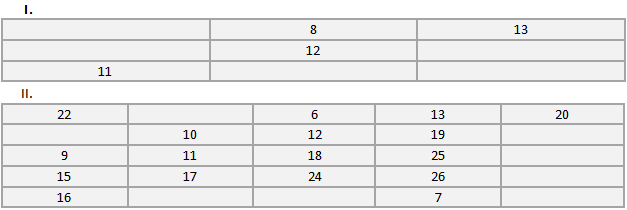Solution 1:

I.  If we see that in diagonal pattern, 13 + 12 + 11 = 36.

Thus,

Number in the first cell of the first row = 36 – (8 + 13) = 15

Number in the first cell of the second row = 36 – (15 + 11) = 10

Number in the third cell of the second row = 36 – (10 + 12) = 14

Number in the second cell of the third row = 36 – (8 + 12) = 16

Number in the third cell of the third row = 36 – (11 + 16) = 9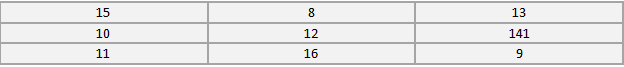II.  If we see that in diagonal pattern, 20 + 19 + 18 + 17 + 16 = 90.

Thus,

Number in the second cell of the first row = 90 – (22 + 6 + 13 + 20) = 29

Number in the first cell of the second row = 90 – (22 + 9 + 15 + 16) = 28

Number in the fifth cell of the second row = 90 – (28 + 10 + 12 + 19) = 21

Number in the fifth cell of the third row = 90 – (9 + 11 + 18 + 25) = 27

Number in the fifth cell of the fourth row = 90 – (15 + 17 + 24 + 26) = 8

Number in the second cell of the fifth row = 90 – (29 + 10 + 11 + 17) = 23

Number in the third cell of the fifth row = 90 – (6 + 12 + 18 + 24) = 30Question 2:  Perform the following subtractions and check your results by performing corresponding additions:

i.        57839 – 29883

ii.        92507 – 10879

iii.        400000 – 98798

iv.        5050501 – 969696

v.        200000 – 97531

vi.        3030301 – 868686

Solution 2: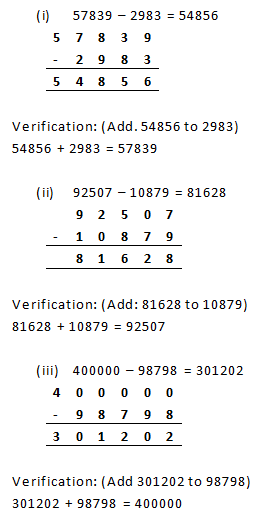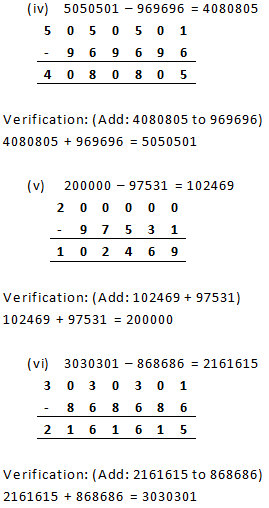Question 3:  Replace each * by the correct digit in each of the following: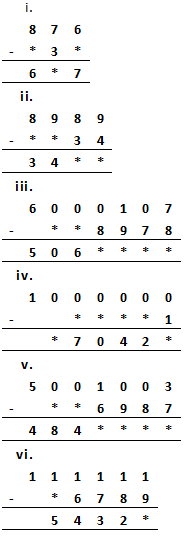Solution 3:

(i)   Here, we see that in the once place, 6 – * = 7, which means that the value of * is 9, as 1 borrow from 7 at tens place to 6 at once place and 6 at once digit becomes 16 then 16 – 9 = 7.Now, tens place  the value is 6 ,  so 6 – 3 = 3.

Also, it can be easily deduced that in (8 – * = 6), the value of * is 2.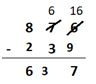(ii) Here, it is clear that in the once place, 9 – 4 = 5; And in the tens place, 8 – 3 = 5.

We can now easily find out the other missing blanks by subtracting 3455 from 8989. Addend (difference) = 3455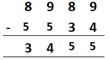(iii) Here, in the units digit, 17 – 8 = 9; in the tens digit, 9 – 7 = 2;

in the hundreds place, 10 – 9 = 1;

and in the thousands place, 9 – 8 = 1.

So, in order to get the addend, we will subtract 5061129 from 6000107.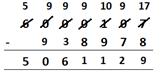(iv) In the once place, 10 -1 = 9;

Also, in the lakhs place, 9 -0 = 9;

So, in order to get the addend, we will subtract 970429 from 1000000.(v) Here, in the once digit, 13 – 7 = 6;

in the tens digit, 9 – 8 = 1;

in the hundreds place, 9 – 9 = 0;

and in the thousands place, 10 – 6 = 4.

So, in order to get the addend, we will subtract 4844016 from 5001003.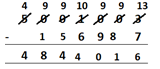(vi) It is clear from the once place that 11 – 9 = 2.

To get the other addend, we will subtract 54322 from 111111.

Thus, the other addend is 56789.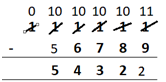Question 4:  What is the difference between the largest number of five digits and smallest number of six digits?

Solution 4:

The largest 5 – digit number is 99999.

The smallest 6 – digit number is 100000.

Therefore, difference between the largest 5 – digit number and smallest 6 – digit number

= 100000 – 99999 = 1

Question 5:  Find the difference between the largest number of 4 digits and the smallest number of 6 digits.

Solution 5:

The largest 4 – digit number is 9999.

The smallest 7 – digit number is 1000000.

Therefore, difference between the largest 4 – digit number and smallest 7 – digit number

= 1000000 – 9999 = 990001

Question 6:  Rohit deposited Rs 125000 in his savings bank account. Later he withdrew Rs 35425 from it. How much money was left in his account?

Solution 6:

Money deposited by Rohit = Rs 125000

Money withdrawn by Rohit = Rs 35425

Therefore, left money in the account = Rs. (125000 – 35425) = Rs 89575

Question 7:  The population of a town is 96209. If the number of men is 29642 and that of women is 29167, determine the number of children.

Solution 7:

Total population of the town = 96209

Number of men = 29642

Number of women = 29167

Sum of men and women = (29642 + 29167) = 58809

Therefore, Number of children in the town = (Total population) – (Sum of men and women)

= 96209 – 58809 = 37400

Question 8:  The digits of 6 and 9 of the number 36490 are interchanged. Find the difference between the original number and the new number.

Solution 8:

Original number = 39460

New number = 36490 (after interchange the place value of 6 and 9 )

Difference = 39460 – 36490 = 2970

Question 9:  The population of a town was 59000. In one year, it was increased by 4563 due to new births. However, 9218 persons died or left the town during the year. What was the population at the end of the year?

Solution 9:

Population of the town = 59000

Increase in the population = 4536

Decrease in the population = 9218

New population = 59000 + 4536 – 9218 = 54318

Exercise 4.3

Question 1:  Fill in the blanks to make each of the following a true statement:

(i) 785 × 0 = _____

(ii) 4567 × 1 = _____

(iii) 475 × 129 = 129 × _____

(iv) _____ × 8975 = 8975 × 1243

(v) 10 × 100 × _____ = 10000

(vi) 27 × 18 = 27 × 9 + 27 × _____ + 27 × 5

(vii) 12 × 45 = 12 × 50 – 12 × _____

(viii) 78 × 89 = 78 × 100 – 78 × _____ + 78 × 5

(ix) 66 × 85 = 66 × 90 – 66 × _____ – 66

(x) 49 × 66 + 49 × 34 = 49 × (_____+ _____)

Solution 1:

(i) 785 × 0 = 0

(ii) 4567 × 1 = 4567 (Multiplicative identity)

(iii) 475 × 129 = 129 × 475 (Commutativity)

(iv) 1243 × 8975 = 8975 × 1243 (Commutativity)

(v) 10 × 100 × 10 = 10000

(vi) 27 × 18 = 27 × 9 + 27 × 4 + 27 × 5

(vii) 12 × 45 = 12 × 50 – 12 × 5

(viii) 78 × 89 = 78 × 100 – 78 × 16 + 78 × 5

(ix) 66 × 85 = 66 × 90 – 66 × 4 – 66

(x) 49 × 66 + 49 × 34 = 49 × (66 + 34)

Question 2:  Determine each of the following products by suitable rearrangements:

(i) 2 × 1497 × 50

(ii) 4 × 358 × 25

(iii) 495 × 625 × 16

(iv) 625 × 20 × 8 × 50

Solution 2:

(i) 2 × 1497 × 50

= (2 × 50) × 1497

= 100 × 1497

= 149700

(ii) 4 × 358 × 25

= (4 × 25) × 358

= 100 × 358

= 35800

(iii) 495 × 625 × 16

= (625 × 16) × 495

= 10000 × 495

= 4950000

(iv) 625 × 20 × 8 × 50

= (625 × 8) × (20 × 50)

= 5000 × 1000

= 5000000

Question 3:  Using distributivity of multiplication over addition of whole numbers, find each of the following products:

(i) 736 × 103

(ii) 258 × 1008

(iii) 258 × 1008

Solution 3:

(i) 736 × 103 = 736 × (100 + 3)

{Using distributivity of multiplication over addition of whole numbers}

= (736 × 100) + (736 × 3)

= 73600 + 2208 = 75808

(ii) 258 × 1008 = 258 × (1000 + 8)

{Using distributivity of multiplication over addition of whole numbers}

= (258 × 1000) + (258 × 8)

= 258000 + 2064 = 260064

(iii) 258 × 1008 = 258 × (1000 + 8)

{Using distributivity of multiplication over addition of whole numbers}

= (258 × 1000) + (258 × 8)

= 258000 + 2064 = 260064

Question 4:  Find each of the following products:

(i) 736 × 93

(ii) 816 × 745

(iii) 2032 × 613

Solution 4:

(i) 736 × 93

Since, 93 = (100 – 7)

Therefore, 736 × (100 – 7)

= (736 × 100) – (736 × 7)

(Using distributivity of multiplication over subtraction of whole numbers)

= 73600 – 5152 = 68448

(ii) 816 × 745

Since, 745 = (750 – 5)

Therefore, 816 × (750 – 5)

= (816 × 750) – (816 × 5)

(Using distributivity of multiplication over subtraction of whole numbers)

= 612000 – 4080 = 607920

(iii) 2032 × 613

Since, 613 = (600 +13)

Therefore, 2032 × (600 + 13)

= (2032 × 600) + (2032 × 13)

= 1219200 + 26416 = 1245616

Question 5:  Find the values of each of the following using properties:

(i) 493 × 8 + 493 × 2

(ii) 24579 × 93 + 7 × 24579

(iii) 1568 × 184 – 1568 × 84

(iv) 15625 × 15625 – 15625 × 5625

Solution 5:

(i) 493 × 8 + 493 × 2

= 493 × (8 + 2)

(Using distributivity of multiplication over addition of whole numbers)

= 493 × 10 = 4930

(ii) 24579 × 93 + 7 × 24579

= 24579 × (93 + 7)

(Using distributivity of multiplication over addition of whole numbers)

= 24579 × 100 = 2457900

(iii) 1568 × 184 – 1568 × 84

= 1568 × (184 – 84)

(Using distributivity of multiplication over subtraction of whole numbers)

= 1568 × 100 = 156800

(iv) 15625 × 15625 – 15625 × 5625

= 15625 × (15625 – 5625)

(Using distributivity of multiplication over subtraction of whole numbers)

= 15625 × 10000 = 156250000

Question 6:  Determine the product of:

(i) the greatest number of four digits and the smallest number of three digits.

(ii) the greatest number of five digits and the greatest number of three digits.

Solution 6:

(i) The largest four-digits number = 9999

The smallest three – digits number = 100

Therefore, Product of the smallest three-digit number and the largest four-digit number = 9999 × 100 = 999900

(ii) The largest five – digits number = 9999

The largest number of three digits = 999

Therefore, Product of the largest three-digit number and the largest five-digit number

= 9999 × 999

= 9999 × (1000 — 1)

= (9999 × 1000) — (9999 × 1)

= 9999000 – 9999

= 9989001

Question 7:  In each of the following, fill in the blanks, so that the statement is true:

`i.               (500 + 7) (300 - 1) = 299 × _____`
`ii.              888 + 777 + 555 = 111 × _____`
`iii.            75 × 425 = (70 + 5) (_____+ 85)`
`iv.            89 × (100 - 2) = 98 × (100 - _____)`
`v.             (15 + 5) (15 - 5) = 225 - _____`
`vi.            9  × (10000 + _____)= 98766 `

Solution 7:

(i)(500 + 7) (300 – 1)

= 507 × 299

= 299 × 507 (By Using Commutativity)

(ii) 888 + 777 + 555

= 111 (8 + 7 + 5)

= 111 × 20 (By Using Distributivity)

(iii) 75 × 425

= (70 + 5) × 425

= (70 + 5) (340 + 85)

(iv) 89 × (100 – 2)

= 89 × 98

= 98 × 89

= 98 × (100 – 11) (By Using Commutativity)

(v) (15 + 5) (15 – 5)

= 20 × 10

= 200

= 225 – 25

(vi) 9 × (10000 + 974)

= 98766

Question 8:  A dealer purchased 125 color television sets. If the cost of each set is Rs 19820, determine the cost of all sets together.

Solution 8:

Cost of 1 color television set = Rs. 19820

Therefore, Cost of 125 color television sets

= Rs (19820 × 125)

= Rs 19820 × (100 + 25)

= Rs (19820 × 100) + (19820 × 25)

= Rs 1982000 + 495500

= Rs 2477500

Question 9:  The annual fee charged from a student of class 6th in a school is Rs 8880. If there are, in all, 235 students in class 6th, find the total collection.

Solution 9:

Fees charged from 1 student = Rs. 8880

Therefore, Fees charged from 235 students

= Rs. 8880 × 235

= Rs. 2086800

Thus, the total collection from class VI students is Rs 2086800.

Question 10:  A group housing society constructed 350 flats. If the cost of construction for each flat is Rs 993570, what is the total cost of construction of all the flats.

Solution 10:

Cost of construction of 1 flat = Rs. 993570

Total number of flats constructed = 350

Total cost of construction of 350 flats

= Rs. (993,570 × 350)

= Rs. 347,749,500

Question 11:  The product of two whole numbers is zero. What do you conclude?

Solution 11:   If the product of two whole numbers is zero, then it means that either one of them is zero or both of them are zero.

Question 12:  What are the whole numbers which when multiplied with itself gives the same number?

Solution 12:   There are two whole numbers which when multiplied with themselves give the same numbers.

Examples:

(i) 0 × 0 = 0

(ii) 1 × 1 = 1

Question 13:  In a large housing complex, there are 15 small buildings and 22 large building. Each of the large buildings has 10 floors with 2 apartments on each floor. Each of the small buildings has 12 floors with 3 apartments on each floor. How many apartments are there in all.

Solution 13:

Given:

Number of large buildings = 22

Number of small buildings = 15

Number of floors in 1 large building = 10

Number of apartments on 1 floor = 2

Therefore, Total apartments in 1 large building are = 10 × 2 = 20

Similarly,

Total apartments in 1 small building are = 12 × 3 = 36

Therefore, Total apartments in the entire housing complex

= (22 × 20) + (15 × 36)

= 440 + 540

= 980

Exercise 4.4

Question 1:  Does there exists a whole number ‘a’ such that a÷a = a?

Solution 1:

Yes, there exists a whole number ‘a’ such that a÷a = a.

The whole number is 1 such that,

1÷1 = 1

Question 2:  Find the value of:

(i) 23457 ÷ 1

(ii) 0 ÷97

(iii) 476 + (840 ÷ 84)

(iv) 964 – (425 ÷ 425)

(v) (2758 ÷ 2758) – (2758 + 2758)

(vi) 72450 ÷ (583 – 58)

Solution 2:

(i) 23457 ÷ 1 = 23457

(ii) 0 ÷97 = 0

(iii) 476 + (840 ÷ 84) = 476 + 10 = 486

(iv) 964 – (425 ÷ 425) = 964 – 1 = 963

(v) (2758 ÷ 2758) – (2758 + 2758) = 1 – 1 = 0

(vi) 72450 ÷ (583 – 58) = 72450 + 525 = 138

Question 3:  Which of the following statements are true:

i.          10 ÷ (5 × 2) = (10 ÷ 5) × (10 ÷ 2)

ii.        (35 – 14) ÷ 7 = 35 ÷ 7 – 14 ÷ 7

iii.       35 – 14 ÷ 7 = 35 ÷ 7 – 14 ÷ 7

iv.       (20 - 5) ÷5 = 20 ÷ 5 – 5

v.        12 × (14 ÷ 7) = (12 ×14) ÷ (12× 7)

vi.       (20 ÷ 5) ÷ 2 = (20 ÷ 2) ÷ 5

Solution 3:

(i) False

LHS: 10 ÷ (5 × 2)

= 10 ÷ 10

=1

RHS: (10 ÷5) × (10 ÷ 2)

= 2 × 5 = 10

(ii) True

LHS: (35 – 14) ÷ 7

= 21 ÷ 7

=3

RHS: 35 ÷ 7 – 14 ÷ 7

= 5 – 2 = 3

(iii) False

LHS: 35 – 14 ÷ 7

= 35 – 2 = 33

RHS: 35 ÷ 7 – 14 ÷ 7

= 5 – 2

= 3

(iv) False

LHS: (20 – 5) ÷ 5

= 15 ÷ 5

= 3

RHS: 20 ÷ 5 – 5

= 4 – 5 = -1

(v) False

LHS: 12 × (14 ÷ 7)

= 12 × 2

= 24

RHS: (12 × 14) ÷ (12 × 7)

= 168 ÷ 84

=2

(vi) True

LHS: (20 ÷ 5) ÷ 2

= 4 ÷ 2

=2

RHS: (20 ÷ 2 ) ÷ 5

= 10 ÷ 5

= 2

Question 4:  Divide and check the quotient and remainder:

i. 7772 ÷ 58

ii.6906 ÷ 35

iii.16135 ÷ 875

iv. 16025 ÷ 1000

Solution 4: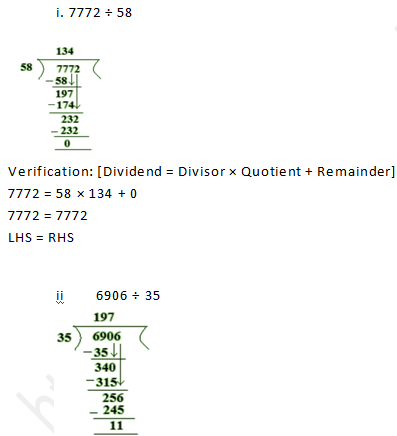Verification: [Dividend = Divisor × Quotient + Remainder]

6906 = 35 × 197 + 11

6906 = 6895 + 11

6906 = 6906

LHS = RHSQuestion 5:  Find a number which when divided by 35 gives the quotient 20 and remainder 18.

Solution 5:

Dividend = Divisor × Quotient + Remainder

Dividend = 35 × 20 + 18

= 700 + 18

= 718

Question 6:  Find the number which when divided by 58 gives a quotient 40 and remainder 31.

Solution 6:

Dividend = Divisor × Quotient + Remainder

Dividend = 58 × 40 + 31

= 2320 + 31

= 2351

Question 7:  The product of two numbers is 504347. If one of the numbers is 1591, find the other.

Solution 7:

Product of two numbers = 504347

One of the two numbers = 1591

Let the number be a.

Therefore, a × 1591 = 504347Question 8:  On dividing 59761 by a certain number, the quotient is 189 and the remainder is 37. Find the divisor.
Solution 8:
Dividend = 59761
Quotient = 189
Remainder = 37
Divisor = a
Now, Dividend = Divisor × Quotient + Remainder
59761 =a × 189 + 37
59761 – 37 = a × 189
59724 = a × 189

Therefore, a = 59724/189
Divisor = a = 316

Question 9:  On dividing 55390 by 299, the remainder is 75. Find the quotient.

Solution 9:

Dividend = 55390

Divisor = 299

Remainder = 75

Quotient = a

Dividend = Divisor × Quotient + Remainder

55390 = 299 × a + 75

55390 – 75 = a × 299

55315 = a × 299

Therefore, a = 55315/299

Quotient = a = 185

Exercise 4.5

Question 1:  Without drawing a diagram, find:

Solution 1:

(i) 10th square number:

Rule for remembering a square number :

Nth square number = n × n

Therefore, 10th square number = 10 × 10 = 100

(ii) 6th triangular number:

Role from remembering a triangular number

Nth triangular number = n × (n + 1 )2

Therefore, 6th triangular number = 6 × (6 + 1)2 = 21

Question 2:

(i) Can a rectangle number also be a square number?

(ii) Can a triangular number also be a square number?

Solution 2:

(i)     Yes, a rectangular number can also be a square number;

Here we take an example, 16 is a square number also a rectangular number.Question 3:   Write the first four products of two numbers with difference 4 starting from in the following order:

1 , 2 , 3 , 4 , 5 , 6 , ………..

Identify the pattern in the products and write the next three products.

Solution 3:

1 × 5 = 5

2 × 6 = 12

3 × 7 = 21

4 × 8 = 32

Therefore, the first 4 products of two number with difference 4:

5 – 1 = 4

6 – 2 = 4

7 – 3 = 4

8 – 4 = 4

Question 4:  Observe the pattern in the following and fill in the blanks:

9 × 9 + 7 =88

98 × 9 + 6 = 888

987 × 9 + 5 = 8888

9876 × 9 + 4 = _____

98765 × 9 + 3 = _____

987654 × 9 + 2 = _____

9876543 × 9 + 1 = _____

Solution 4:

9 × 9 + 7 =88

98 × 9 + 6 = 888

987 × 9 + 5 = 8888

9876 × 9 + 4 = 88888

98765 × 9 + 3 = 888888

987654 × 9 + 2 = 8888888

9876543 × 9 + 1 = 88888888

Question 5:  Observe the following pattern and extend it to three more steps:

6 × 2 – 5 = 7

7 × 3 – 12 = 9

8 × 4 – 21 = 11

9 × 5 – 32 = 13

__ × __ – __ = __

__ × __ – __ = __

__ × __ – __ = __

Solution 5:

6 × 2 – 5 = 7

7 × 3 – 12 = 9

8 × 4 – 21 = 11

9 × 5 – 32 = 13

10 × 6 – 45 = 15

11 × 7 – 60 = 17

12 × 8 – 77 = 19

Question 6:  Study the following pattern:

1 + 3 = 2 × 2

1 + 3 + 5 = 3 × 3

1 + 3 + 5 + 7 = 4 × 4

1 + 3 + 5 + 7 + 9 = 5 × 5

By observing the above pattern, find:

(i) 1 + 3 + 5 + 7 + 9 + 11

(ii) 1 + 3 + 5 + 7 + 9 + 11 + 13 + 15

(iii) 21 + 23 + 25 + … + 51

Solution 6:

(i) 1 + 3 + 5 + 7 + 9 + 11

= 6 × 6

= 36

(ii) 1 + 3 + 5 + 7 + 9 + 11 + 13 + 15

= 8 × 8

= 64

(iii) 21 + 23 + 25 + … + 51

= (21 + 23 + 25 +…+ 51) can also be written as

(1 + 3 + 5 + 7 +…+ 49 + 51) – (1 + 3 + 5 +…+ 17 + 19)

(1 + 3 + 5 + 7+…+ 49 + 51) = 26 × 26 = 676

and, (1 + 3 + 5 +…+ 17 + 19 ) = 10 × 10 = 100

Now,

(21 + 23 + 25 +…+ 51 ) =  676  – 100 = 576

Question 7:  Study the following pattern: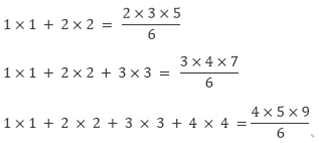By observing the above pattern, write next two steps.

Solution 7:

The next two steps are as follows: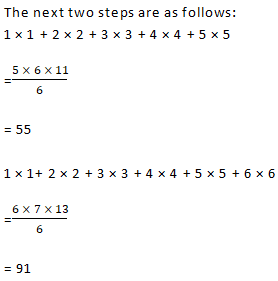Question 8:  Study the following pattern:By observing the above pattern, find:

(i) 1 + 2 + 3 + 4 + 5 + 6 + 7 + 8 + 9 + 10

(ii) 50 + 51 + 52 + …+ 100

(iii) 2 + 4 + 6 + 8 + 10 +…+ 100

Solution 8:

(i) 1 + 2 + 3 + 4 + 5 + 6 + 7 + 8 + 9 + 10

= (10 × 11)/2

= 55

(ii) 50 + 51 + 52 + …+ 100

We can also be written as

=(1 + 2 + 3 + …+ 99 + 100) – (1 + 2 + 3 + 4 + …+ 47 + 49)

Now,

=(1 + 2 + 3 + …+ 99 + 100 ) = (100 × 101)/2

and, (1 + 2 + 3 + 4 +…+ 47 + 49 ) = (49 × 50)/2

So, (50 + 51 + 52 + …+ 100 ) = (100 × 101)/2 – (49 × 50)/

2

= 5050 – 1225

= 3825

(iii) 2 + 4 + 6 + 8 + 10 +…+ 100

We can also be written as

2 × (1 + 2 + 3 + 4 + …+ 49 + 50)

Now,

(1 + 2 + 3 + 4 + …+ 49 + 50 ) = 50 × 512

= 1275

Therefore, (2 + 4 + 6 + 8 + 10 + …+ 100) = 2 × 1275 = 2550

Exercise 4.6

Question 1:  Which one of the following is the smallest whole number?

(a) 1  (b)  2   (c) 0  (d) None of these

Solution 1:

The set of whole numbers is {0 , 1, 2, 3, 4, …}.

So, the smallest whole number is 0.

Question 2:  Which one of the following is the smallest even whole number?

(a) 0 (b) 1 (c) 2 (d) None of these

Solution 2:

The natural numbers along with 0 form the collection of whole numbers.

So, the numbers 0, 1, 2, 3, 4, … form the collection of whole numbers.

The number which is divisible by 2 is an even number.

So, in the collection “0, 1, 2, 3, 4, …”, 2 is the smallest even number.

Question 3:  Which one of the following is the smallest odd whole number?

(a) 0 (b) 1 (c) 3 (d) 5

Solution 3:

The natural numbers along with 0 form the collection of whole numbers.

So, the numbers 0, 1, 2, 3, 4, … form the collection of whole numbers.

A natural number which is not divisible by 2 is called an odd whole number.

So, in the collection “0, 1, 2, 3, 4, …”, 1 is the smallest odd whole number.

Question 4:  How many whole numbers are between 437 and 487?

(a) 50 (b) 49 (c) 51 (d) None of these

Solution 4:

The whole numbers between 437 and 487 are 438, 439, 440, 441, … , 484, 485 and 486. To find the required number of whole numbers,

We need to subtract 437 from 487 and then subtract again 1 from the result.

Thus, there are (487 – 437) – 1 whole numbers between 437 and 487.

Now, (487 – 437) – 1 = 50 – 1 = 49

Question 5:  The product of the successor 999 and predecessor of 1001 is:

(a) one lakh (b) one billion (c) one million (d) one crore

Solution 5:

Successor of 999 = 999 + 1 = 1000

Predecessor of 1001 = 1001 – 1 = 1000

Now,

Product = (Successor of 999) × (Predecessor of 1001)

= 1000 × 1000

= 1000000

= one million

Question 6:   Which one of the following whole numbers does not have a predecessor?

(a) 1 (b) 0 (c) 2 (d) None of these

Solution 6:

The numbers 0, 1, 2, 3, 4, …. form the collection of whole numbers.

0 is the smallest whole number.

So, 0 does not have a predecessor.

Question 7:  The number of whole numbers between the smallest whole number and the greatest 2 digit number is:

(a) 101 (b) 100  (c) 99 (d) 98

Solution 7:

Smallest whole number = 0

Greatest 2-digit whole number = 99

The whole numbers between 0 and 99 are 1, 2, 3, 4 …… 97, 98.

To find the number of whole numbers between 0 and 99,

Subtract 1 from the difference of 0 and 99.

Therefore, Number of whole numbers between 0 and 99 = (99 – 0) – 1

= 99 – 1

= 98

Question 8:  If n is a whole number such that n + n = n, then n =?

(a) 1 (b) 2 (c) 3 (d) None of these

Solution 8:

Here, 0 + 0 = 0, 1 + 1 = 2, 2 + 2 = 4 …..

So, the statement n + n = n is true only when n = 0.

Question 9:  The predecessor of the smallest 3 digit number is:

(a) 999 (b) 99 (c) 100 (d) 101

Solution 9:

Smallest number of 3 – digit = 100

Predecessor of 3-digit number = 100 — 1 = 99

Question 10:  The least number of 4 digits which is exactly divisible by 9 is:

(a)1008  (b)1009  (c)1026  (d)1018

Solution 10:

Least number of 4-digit = 1000

The least 4-digit number exactly divisible by 9 is 1000 + (9 – 1) = 1008.

Question 11:   The number which when divided by 53 gives 8 as quotient and 5 as remainder is:

(a) 424 (b) 419 (c) 429 (d) None of these

Solution 11:

Here, Divisor = 53, Quotient = 8 and Remainder = 5.

Now, using the Relation Dividend = Divisor x Quotient + Remainder

We get

Dividend = 53 x 8 + 5

= 424 + 5

= 429

Thus, the required number is 429.

Question 12:  The whole number n satisfying n + 35 = 101 is:

(a) 65 (b) 67 (c) 64 (d) 66

Solution 12:

Here, n+ 35 = 101.

Adding – 35 on both sides, we get

n + 35 + (- 35) = 101 + (- 35)

n + 0 = 66

n = 66

Question 13:  The value of 4 x 378 x 25 is:

(a) 37800 (b) 3780 (c) 9450 (d) 30078

Solution 13:

By Regrouping, we get

=4 × 378 × 25

= 4 × 25 × 378

= 100 × 378

= 37800

Question 14:   The value of 1735 x 1232 – 1735 x 232 is:

(a) 17350 (b) 173500 (c) 1735000 (d) 173505

Solution 14:

By using Distributive Law of Multiplication over subtraction, we get

=1735 × 1232 – 1735 × 232

= 1735 (1232 – 232)

= 1735 × 1000

= 1735000

Question 15:   The value of 47 × 99 is:

(a) 4635 (b) 4653 (c) 4563 (d) 6453

Solution 15:

Since, 99 = 100 — 1

Therefore, 47 × 99 = 47 × (100 — 1)

= 47 × 100 — 47

= 4700 — 47

= 4653

Thus, the value of 47 × 99 is 4653.